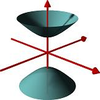#### You may also like### A Close Match

Can you massage the parameters of these curves to make them match as closely as possible?### Prime Counter

A short challenge concerning prime numbers.### The Right Volume

Can you rotate a curve to make a volume of 1?

# Cubic Roots

##### Age 16 to 18 ShortChallenge Level

A certain cubic polynomial $y=f(x)$ cuts the $x$-axis at  the three points $x=10, 100$ and $1000$. Is this enough information to determine the location of its point of inflection (note that this is not necessarily a stationary point of inflection)? If so, where is this point; if not, why not?

Construct a cubic polynomial which cuts the $x$-axis at $x=10, 100$ and its point of inflection. How many such polynomials are there?

Did you know ... ?

Polynomials have many fascinating properties. A key result of university mathematics is the Fundamental Theorem of Algebra which states that any polynomial of degree $n$ $p(z)= a_nz^n+a_{n-1}z^{n-1}+\dots+a_0$, with $a_n\neq 0$, has precisely $n$, possibly repeated, complex number solutions.